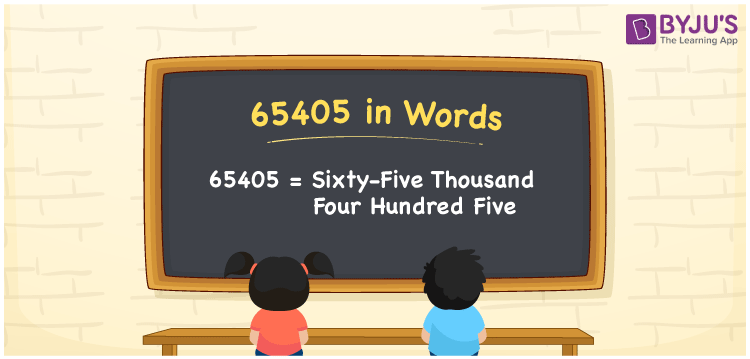# 65405 in Words

We can write 65405 in words as Sixty-five thousand four hundred five. Thus, the number name or word form of 65405 is Sixty-five thousand four hundred five. Also, we use the number name of 65405 to express it to others. In this article, you will learn how to transform the cardinal number 65405 into words in an easily understandable method.

 65405 in words Sixty-five thousand four hundred five Sixty-five thousand four hundred five in Numbers 65405

## 65405 in English Words

We generally express numbers in words using the English alphabet. Thus, we read 65405 in English as “Sixty-five thousand four hundred five”.## How to Write 65405 in Words?

As we can see, 65405 is a five-digit number so let’s create a place value chart with five columns as given below.

 Ten thousands Thousands Hundreds Tens Ones 6 5 4 0 5

From the above, we can write the numbers as:

6 × Ten thousand + 5 × Thousand + 4 × Hundred + 0 × Ten + 5 × One

= 1 × 60000 + 5 × 1000 + 4 × 100 + 0 × 10 + 5 × 1

= 60000 + 5000 + 400 + 5

= Sixty thousand + Five thousand + Four hundred + Five

= Sixty-five thousand four hundred five

Hence, 65405 in words is written as Sixty-five thousand four hundred five.

65405 is a natural number that precedes 65406 and succeeds 65404.

65405 in words – Sixty-five thousand four hundred five

Is 65405 an even number? – No

Is 65405 an odd number? – Yes

Is 65405 a prime number? – No

Is 65405 a composite number? – Yes

Is 65405 a perfect square number? – No

Is 65405 a perfect cube number? – No

## Frequently Asked Questions on 65405 in Words

Q1

### How do you write 65405 in words?

We can write the number 65405 in words as “Sixty-five thousand four hundred five”.
Q2

### How to write 65405 in words on a cheque?

On a cheque, we generally write 65405 in words as Sixty-five thousand four hundred five rupees only.
Q3

### Write the value of 65405 + 13595 in word form.

65405 + 13595 = 79000 Thus, the value of 65405 + 13595, i.e., 79000 is written in word form as Seventy-nine thousand.